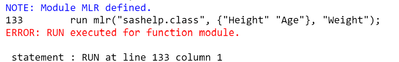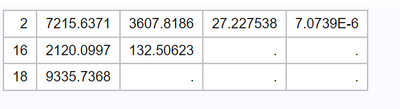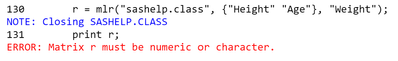## Multiple linear regression using proc iml

I am trying to create a user defined function for multiple linear regression using proc iml. I am trying to create a function where I can input any SAS dataset and values from dataset would be read into two matrices - x for predictors and y for outcome. The matrices would then be used in the formulas for computing estimates for linear regression. But I keep getting an error that dataset doesnt exist. Any suggestions on what is wrong with the code? Also is it possible to input varying number of predictors and still get the function to work?

`proc iml; start linreg(x,y);use dataset;read all var {'x1' 'x2' 'x3'} into x;read all var {'y'} into y;`

1 ACCEPTED SOLUTION

Accepted Solutions

## Re: Multiple linear regression using proc iml

If I understand your question, I believe the answer is yes. The caller would need to specify the COLNAME= and ROWNAME= options.

If the purpose of the module is to display the tables, why not do the printing inside the module? Then you can use the ROWNAME= and COLNAME= options to put nice headers on the output. This is the approach used by the regression module in the SAS/IML Getting Started example, which is very similar to your module.

20 REPLIES 20

## Re: Multiple linear regression using proc iml

What IML code do you intend to use to perform the regression?  It may be that you can create numerical precision issues that PROC REG avoids by default.  Why does this have to be in IML?  Are your users already using IML?

--------------------------
The hash OUTPUT method will overwrite a SAS data set, but not append. That can be costly. Consider voting for Add a HASH object method which would append a hash object to an existing SAS data set

Would enabling PROC SORT to simultaneously output multiple datasets be useful? Then vote for
Allow PROC SORT to output multiple datasets

--------------------------

## Re: Multiple linear regression using proc iml

`proc iml; start mlr(x,y);use dataset;read all var {'x1' 'x2' 'x3'} into x;read all var {'y'} into y;n = nrow(x); /* number of observations */m= ncol(x); /* number of variables */x=j(n,1,1)||x; /* adding a column of 1 corresponding to intercept*//* Compute Xinv, the inverse of X’X and the vector of coefficient estimates Beta. */Computationsfinish mlr;`

This is my code. I cannot understand what is wrong with the code. Any help would be appreciated.

## Re: Multiple linear regression using proc iml

I am not suggesting that there is anything mathematically wrong with your code, but there can be computational issues.

For instance calculating the sums of squares and cross products can be exposed to numeric precision issues for large datasets. Procedures like PROC REG often take a preliminary sample mean from the variables, then get SSCP of the "demeaned" data, and then add back the SSCP component attributable to the means, yielding a more accurate final SSCP than X'X.

This can also happen if there are large scale differences in your variables.

But if your dataset is not large, you are unlikely to have such problems.

--------------------------
The hash OUTPUT method will overwrite a SAS data set, but not append. That can be costly. Consider voting for Add a HASH object method which would append a hash object to an existing SAS data set

Would enabling PROC SORT to simultaneously output multiple datasets be useful? Then vote for
Allow PROC SORT to output multiple datasets

--------------------------

## Re: Multiple linear regression using proc iml

You are not running any IML code here, all you are doing is defining a code module called 'mlr'.  I suggest moving the USE and the two READ statements to the end of the program, after the finish statement, and then after that call the code module using

``run mlr(x, y);``

If you do this, it may work or at least give you an error that can be diagnosed.

## Re: Multiple linear regression using proc iml

Ian's idea is good. If you want to pass in the NAMES of a data set and the NAMES of variables, an alternate syntax is this:

``````proc iml;
/* INPUT:
dsName is a string that specifies the SAS data set. Ex: "sashelp.class"
xNames is a character vector that names the explanatory variables
yName is a character string that names the response variable
*/
start mlr(dsName, xNames, yName);
use (dsName);
read all var xNames into x;
read all var yName into y;
close;

n = nrow(x); /* number of observations */
m= ncol(x); /* number of variables */
x=j(n,1,1)||x; /* adding a column of 1 corresponding to intercept*/
/* ETC. CONTINUE WITH YOUR COMPUTATIONS HERE  */
finish mlr;

run mlr("sashelp.class", {"Height" "Age"}, "Weight");
``````

## Re: Multiple linear regression using proc iml

Thanks! I modified the code but it still gives me this error. I am trying to create a function where I can input any dataset and if I call the function it would still give the output. I am confused as to what is wrong with my code.

`proc iml; INPUT:   dsName = {dataset};   xNames = {'x1' 'x2' 'x3'};   yName ={'y'};start mlr(dsname,xNames,yName);use (dsName);read all var xNames into x;read all var yName into y;close; n = nrow(x); /* number of observations */m= ncol(x); /* number of variables */x=j(n,1,1)||x; /* adding a column of 1 corresponding to intercept*//* Compute Xinv, the inverse of X’X and the vector of coefficient estimates Beta. */xinv=inv(x`*x);beta= xinv*x`*y;finish mlr;run mlr("sashelp.class", {"Height" "Age"}, "Weight");quit;`## Re: Multiple linear regression using proc iml

If you have a function module which returns a value, then you must assign the result to a variable as follows:

``````r = mlr("sashelp.class", {"Height" "Age"}, "Weight");
print (r\$1);``````

In your code lines 2 to 5 are not doing any useful and should be removed.

## Re: Multiple linear regression using proc iml

Just to clarify the call you should be making to analyze you own data should be something like:

``r = mlr("dataset", {"x1" "x2" "x3"}, "y");``

## Re: Multiple linear regression using proc iml

Thank you! It gave me a result but it only gave me the output for first matrix Analysis_of_variance. Is it possible to get results for all the matrices using return? They are in my result matrix but they didnot get output. This is the output I got.## Re: Multiple linear regression using proc iml

I tried print r to print all the components of r but it gave this error.## Re: Multiple linear regression using proc iml

I guess you are running PROC IML at SAS 9.4. In SAS 9.4, the PRINT statement requires a matrix. To print a list, you need to load and use the ListPrint module. See the documentation.

In SAS Viya, the PRINT statement can print lists.

## Re: Multiple linear regression using proc iml

Thank you! I am new to iml and so I am having a bit trouble understanding the syntax. I looked at the documentation and modified the code accordingly but it gave me this error.&nbsp;
proc iml;
start mlr(dsname,xNames,yName);
use (dsName);
read all var xNames into x;
read all var yName into y;
close;

n = nrow(x); /* number of observations */
m= ncol(x); /* number of variables */
x=j(n,1,1)||x; /* adding a column of 1 corresponding to intercept*/

/* Compute Xinv, the inverse of X’X */
return ( result );
finish mlr;

r = mlr("sashelp.class", {"Height" "Age"}, "Weight");
print r;
quit;

## Re: Multiple linear regression using proc iml

Almost correct. Use the syntax you were previously using to assign the list:

result = [Analysis_of_variance, Model_fit, Parameter_estimates,y,yhat,resid];

## Re: Multiple linear regression using proc iml

Thank you! I just had one more question. I understand that when we want to print results within iml we can use colname and rowname to give row and column headings. But that is not possible when we are defining a user defined function using return statement?

From The DO Loop